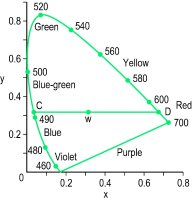# chromaticity diagram

Also found in: Dictionary, Encyclopedia, Wikipedia.

## chromaticity diagram

Plane diagram showing the results of mixtures of colour stimuli, each chromaticity being represented by a single point on the diagram (Fig. C10). Syn. colour triangle. See MacAdam ellipses; CIE standard illuminants; white light; purple; spectrum locus.Fig. C10 The CIE chromaticity diagram (1931) (W, white light illuminant C; C and D, complementary wavelengths)
Millodot: Dictionary of Optometry and Visual Science, 7th edition. © 2009 Butterworth-Heinemann
References in periodicals archive ?
To produce a mixed white light with a color temperature of around 4000 K with phosphor Y-561 and the blue LED, the concentration of phosphors was calculated from the intersection coordinates of the two lines on the CIE chromaticity diagram: the first being the locus of blackbody radiation at 4000 K; the other line being the connection points between the blue LED spectrum and the Y-561 phosphor emission spectrum.
The inset in Figure 1(a) shows the corresponding CIE chromaticity diagram. The chromaticity of the solutions changed as a function of pH (inset in Figure 1(a); pH 3.2: x = 0.318, y = 0.346; pH 12.0: x = 0.304, y = 0.372).
Figure 10(b) shows the CIE chromaticity diagram for 20mol% [Yb.sup.3+]/2mol% [Er.sup.3+] codoped NaY[F.sub.4] microcrystal under the excitation wavelength of 980 nm.
Error ellipse (visual limits): The plotted limits of variation in visually indistinguishable color coordinate values that form an elliptical shape around a central color value when plotted in a chromaticity diagram.
We chose the CIE L*u*v* space (and their corresponding chromaticity diagram CIE u'v') because of the easy psychophysical comprehensibility of their dimensions (see Hunt, 1995).
The reflective spectra are converted into tristimulus XYZ values and then transform the XYZ components into chromaticity coordinates xyz, which can be seen more conveniently for the CIE 1931 color space chromaticity diagram .
The chromaticity coordinates of the ZnO:YAG films at 1, 5, and 10wt% on Si substrate are presented in the CIE chromaticity diagram, as shown in Figure 6.
That's why the LRC recommends using two metrics: CRI, which measures how similar a light source renders colors compared to a reference illuminant, and the gamut area index (GAI), which evaluates the relative area enclosed by sample colors on a chromaticity diagram when rendered by the source.
AN ILLUMINANT'S POSITION ON THE CHROMATICITY DIAGRAM
Chromaticity diagrams (Figure 1) after exposure correction were close to chromaticity diagram of standard colour table in a blue field (for the blue the increased colour difference after the correction is result of too much reduction in brightness due to underexposure).
In terms of the chromaticity diagram, such a decrease means a shift outwards from the "blue" (lower left) corner of the diagram.

Site: Follow: Share:
Open / Close